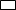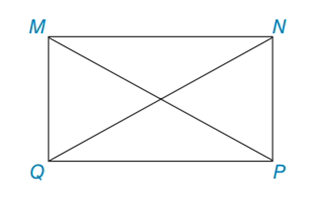Chapter 4.3, Problem 14EElementary Geometry For College St...

7th Edition
Alexander + 2 others
ISBN: 9781337614085

Solutions

Chapter
SectionElementary Geometry For College St...

7th Edition
Alexander + 2 others
ISBN: 9781337614085
Textbook ProblemIn Exercises 11 to 14, consider MNPQ with diagonals M P ¯  and  N Q ¯ . When the answer is not a whole number, leave a square root answer.Exercises 11 – 14If QP = 15 and MP = 17, find MQ and NP.

To determine

To find:

MQ and NP of the given rectangle.

Explanation

The basic property of rectangle:

1) All the properties of a parallelogram apply (the ones that matter here are parallel sides, opposite sides are congruent, and diagonals bisect each other).

2) All angles are right angles by definition.

3) The diagonals are congruent.

Calculation:

Given: QP = 15 and MP = 17

Consider the rectangle.

To find MQ and NP:

Let us take right MQP.

By using Pythagorean Theorem

MP2=MQ2+QP2172=MQ2+

Still sussing out bartleby?

Check out a sample textbook solution.

See a sample solution

The Solution to Your Study Problems

Bartleby provides explanations to thousands of textbook problems written by our experts, many with advanced degrees!

Get Started

Find more solutions based on key concepts